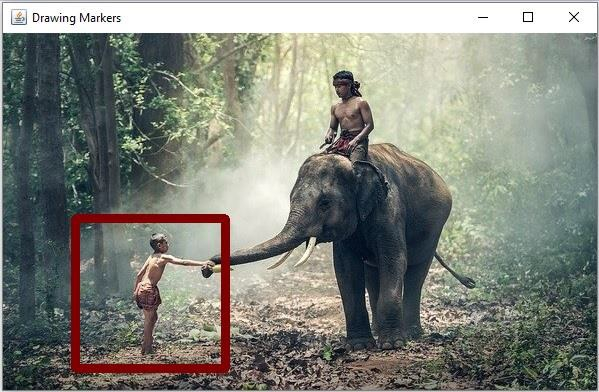# How to draw markers on an image using Java OpenCV library?

You can draw makers on an image using the drawMarker() method of the org.opencv.imgproc.Imgproc class. This method accepts the following parameters −

• img − A Mat object representing the input image.

• position − An object of the class Point to specify the position of the marker.

• color − An object of the class Scalar to specify the color of the marker.

• markerType − An integer constant specifying the type of the marker.

• size − An integer value specifying the size of the marker.

• thickness − An integer value specifying the thickness of the marker.

## Example

import org.opencv.core.Core;
import org.opencv.core.Mat;
import org.opencv.core.Point;
import org.opencv.core.Scalar;
import org.opencv.highgui.HighGui;
import org.opencv.imgcodecs.Imgcodecs;
import org.opencv.imgproc.Imgproc;
public class DrawingMarkers {
public static void main(String args[]) throws Exception {
//Reading the contents of the image
String file ="D:\Images\elephant.jpg";
//Preparing color and position of the marker
Scalar color = new Scalar(0, 0, 125);
Point point = new Point(150, 260);
//Drawing marker
Imgproc.drawMarker(src, point, color, Imgproc.MARKER_SQUARE, 150, 8, Imgproc.LINE_8);
HighGui.imshow("Drawing Markers", src);
HighGui.waitKey();
}
}

## Input Image## Output

On executing the above program generates the following window −